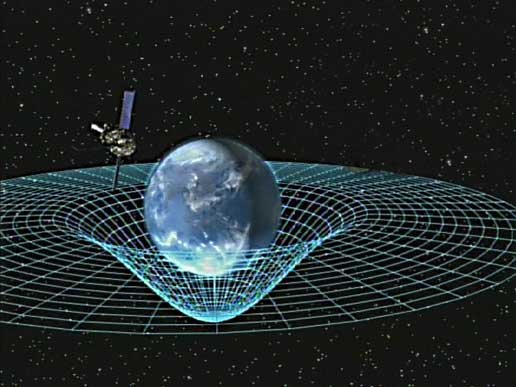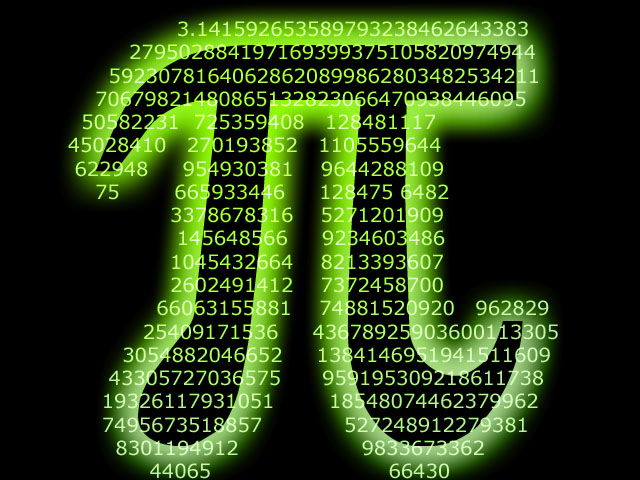#Physics meets Comic-Book Superheroes

The Physics of Superheroes is a popular science book by  James Kakalios, a Physics professor at the School of Physics and Astronomy of the University of Minnesota, and a long-time comic-book fan.  Continue reading “Pow”! “Bang”! The Physics of Superheroes

# The Field Equations of General Relativity# Keeping It Relatively Simple

The Einstein Field Equations of General Relativity are vast and complex, but they can be written with deceptive simplicity.  Using modern notation, the field equations can be formulated as$\boldsymbol G = \frac {- 8 \pi G}{c^4} \boldsymbol T$   Continue reading The Field Equations of General Relativity

#6 Short Videos about the Philosophy of Maths and Science

The Open University has created a series of 6 short animated iTunes videos about the Philosophy behind Maths and Science.   Continue reading 60-Second Adventures in Thought

#12 Short Videos about the Physics of the Cosmos

The Open University has teamed up with “geek chic” comedian David Mitchell to release a series of 12 short animated YouTube videos about the Physics of the Cosmos: “60-Second Adventures in Astronomy”.  A real treat.  And it’s educational!  If you have only 60 seconds, you can now learn everything we know about matter, energy, life, the Universe and everything…

# The Standard Model# 20th Century World View

The Standard Model of Particle Physics is a theory about the electromagnetic, weak and strong nuclear interactions, developed throughout the mid-to-late 20th century, as a worldwide collaborative effort.  Continue reading The Standard Model

#Earth’s Electric Field

The Earth has an electric field.  On average, this field points vertically downwards and it has a magnitude of about 100 N C-1 (Newtons per Coulomb).  It exists because the Earth’s surface carries a negative charge of – 5 x 10C, while the upper atmosphere carries a compensating positive charge.  An average of 400,000 thunderstorms a day  Continue reading Lightning and the Earth’s Electric Field

# Fallen from Outer Space…# Record-Breaking Space Dive

14th October 2012.  During his ultimate high-altitude parachute jump, Felix Baumgartner spends approximately 4 minutes and 22 seconds in freefall, at the maximum speed of 1,342 kilometres per hour.  Continue reading Fallen from Outer Space…

#Physical Constants (SI Units)

Avogadro Constant$N_{A} = 6.022 \times 10^{23} mol^{-1}$

Bohr Radius$a_0 = 5.29 \times 10^{-11} m$

Boltzmann Constant$k = 1.381 \times 10^{-23} JK^{-1}$

Charge on an Electron$e = - 1.602 \times 10^{-19} C$

Gravitational Constant$G = 6.673 \times 10^{-11} Nm^{2} kg^{-2}$

Magnitude of Acceleration due to Gravity$g = 9.81 m s^{-2}$

Permeability of Free Space$\mu_{0} = 4 \pi \times 10^{-7} T m A^{-1}$

Permittivity of Free Space$\epsilon_{0} = 8.854 \times 10^{-12} C^{2} N^{-1} m^{-2}$

Speed of Light in a vacuum$c = 2.998 \times 10^{8} m s^{-1}$